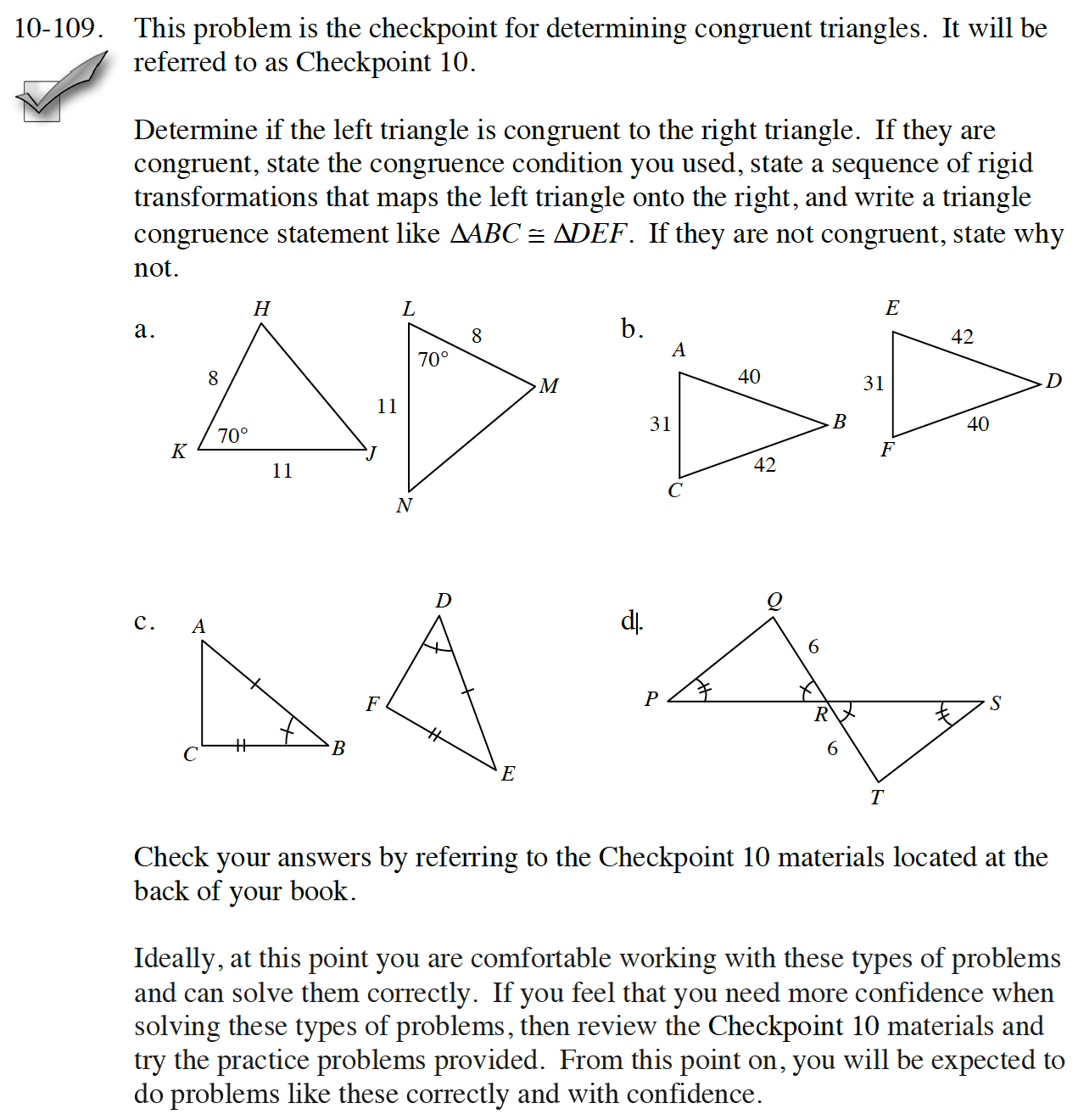### Home > CC4 > Chapter 10 > Lesson 10.2.3 > Problem10-109

10-109.
1.This problem is the checkpoint for determining congruent triangles. It will be referred to as Checkpoint 10. Homework Help ✎

2. Determine if the left triangle is congruent to the right triangle. If they are congruent, state the congruence condition you used, state a sequence of rigid transformations that maps the left triangle onto the right, and write a triangle congruence statement like ∆ABC ≅ ∆DEF. If they are not congruent, state why not.

3.  a.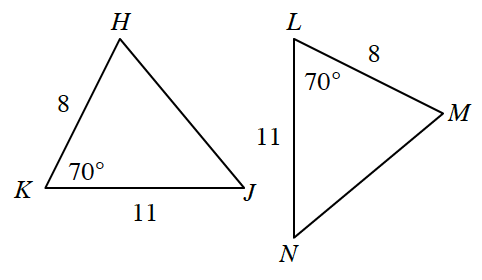b.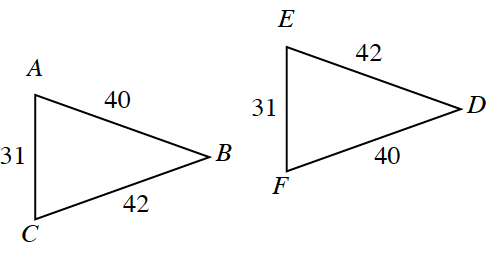c.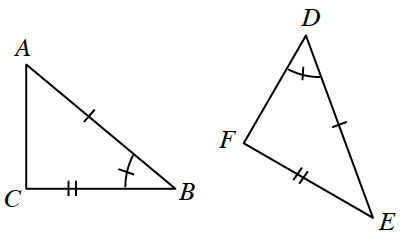d.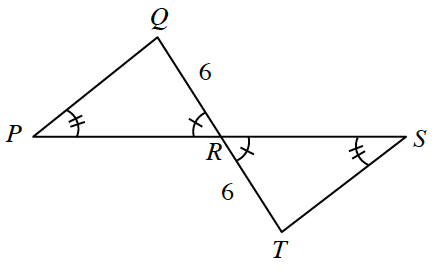​